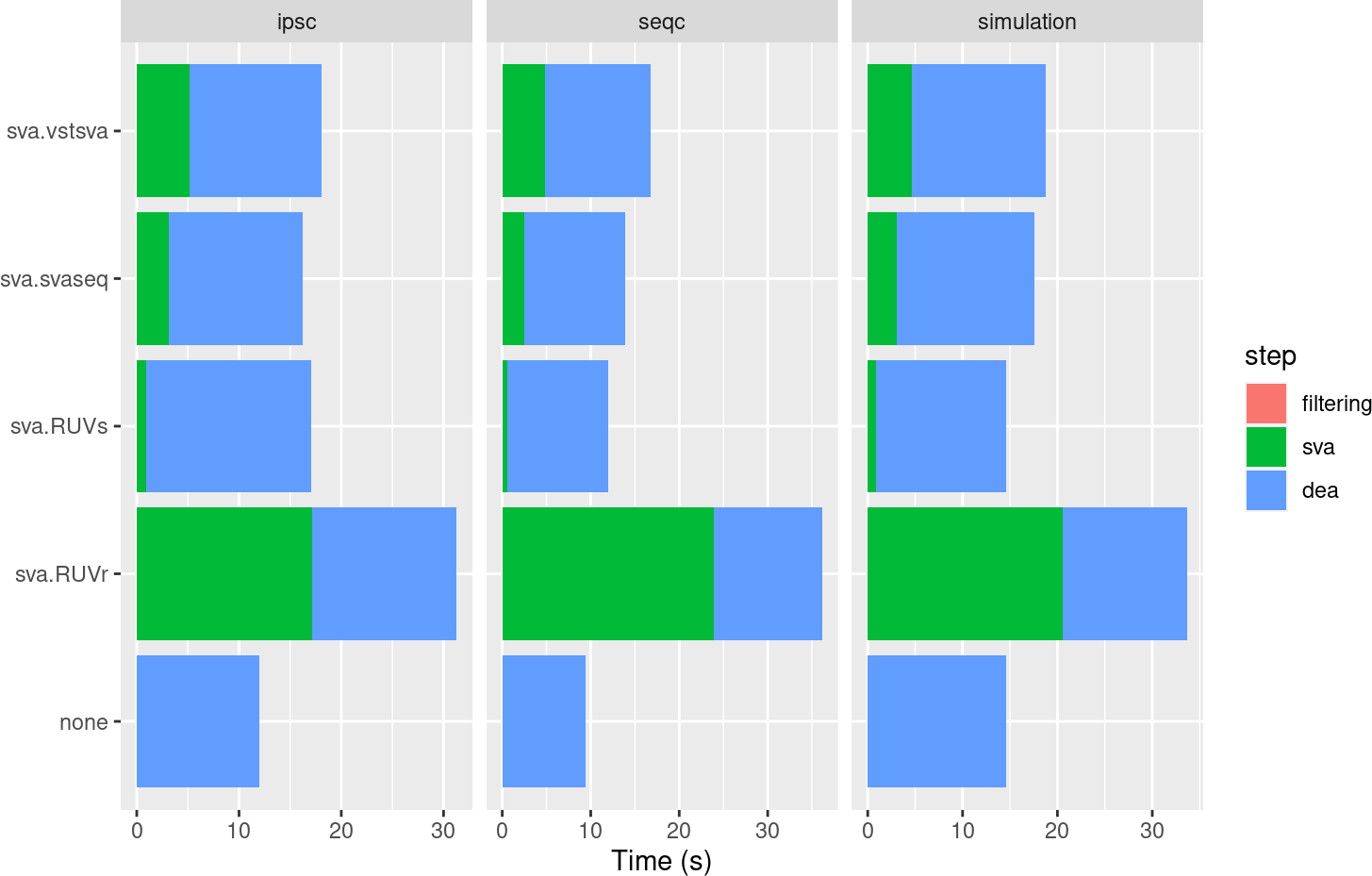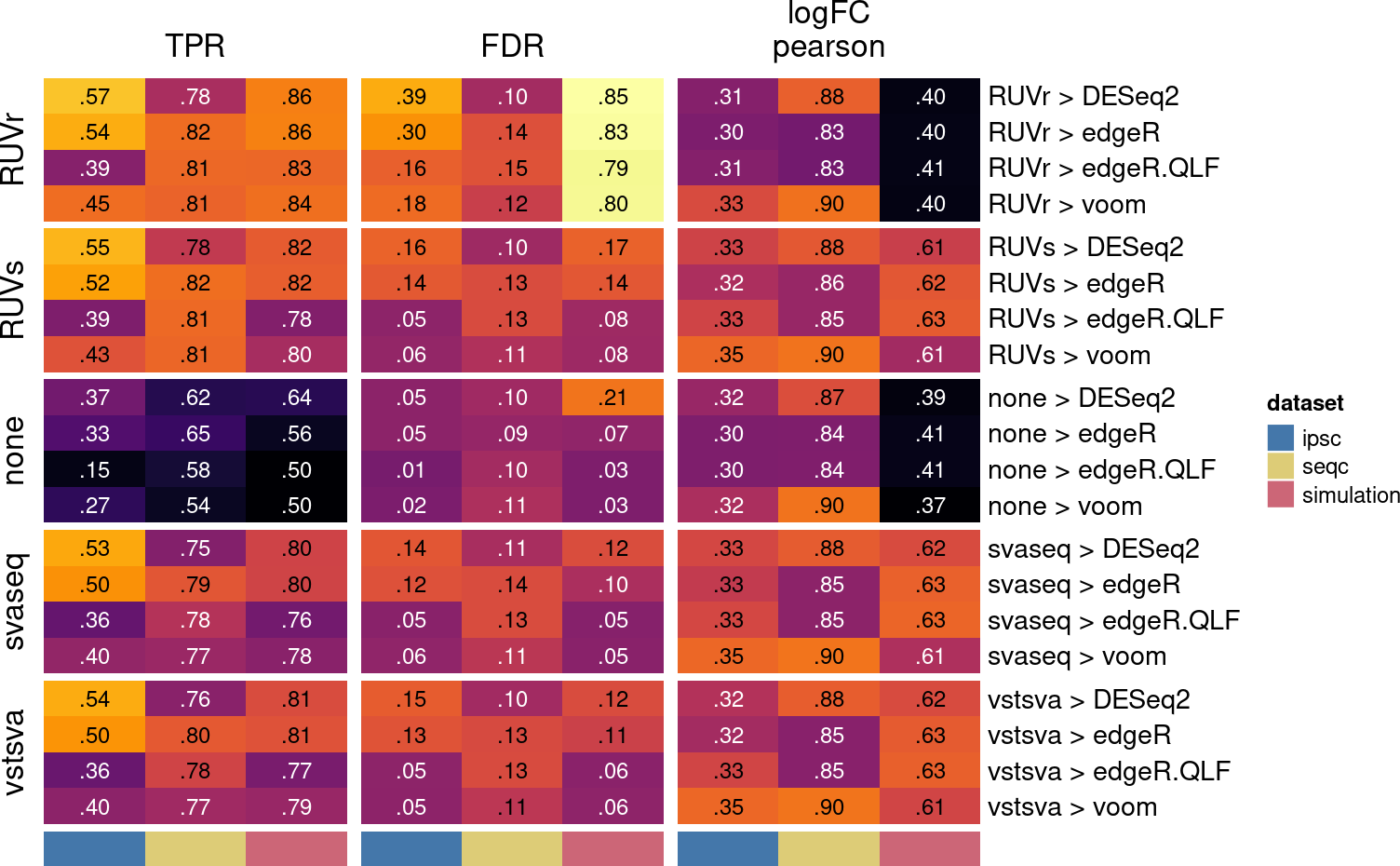# Contents

This vignette is centered around the application of pipeComp to (bulk RNAseq) differential expression analysis (DEA) pipelines involving multiple steps, such as pre-filtering and estimation of technical or unwanted vectors of variation. It will illustrate the whole process of guiding the creating of a new `PipelineDefinition` with a real example. The vignette assumes a general understanding of pipeComp (for an overview, see the pipeComp vignette).

# 1 Introduction

We will create a `PipelineDefinition` which starts with a SummarizedExperiment (see below for more specific requirements) and performs three steps: 1) gene filtering, 2) surrogate variable analysis (SVA) or something analogical (e.g. removal of unwanted variation), and 3) differential expression analysis. The output of the first two steps will be a (filtered, and with the surrogate variables added to ), while the output of the third step will be a differential expression .

We could perform multi-step evaluation, for instance monitoring the genes lost in the filtering step, and the extent to which the SVA-corrected data retains correlation with the technical vectors of variation, but for the sake of simplicity, we will run some evaluation metrics only at the last step.

We will build the `PipelineDefinition` so that the datasets are expected to be objects with a read counts assay (named ‘counts’), a `condition` `colData` factor with two levels, and `rowData` with at least the columns `expected.beta` (expected log2-foldchange) and `isDE` (logical indicating whether the gene is differentially-expressed - NA values are accepted). We’ve prepared three such datasets described in the results section.

# 2 Building the PipelineDefinition object

## 2.1 Preparing evaluation function

We’ll first prepare a function which, given DEA results (as a data.frame), returns evaluation metrics of two kinds: 1) correlation and median absolute error of the estimated foldchanges with respect to the expected ones, and 2) sensitivity, specificity, false discovery rate and such:

``````suppressPackageStartupMessages({
library(pipeComp)
library(S4Vectors)
})

evaluateDEA <- function(dea, truth=NULL, th=c(0.01,0.05,0.1)){
## we make sure that the column names of `dea` are standard:
dea <- pipeComp:::.homogenizeDEA(dea)
## within Pipecomp, the truth should be passed along with the `dea` object, so
## we retrieve it here:
dea <- cbind(dea, truth[row.names(dea),])
## we get rid of genes for which the truth is unknown:
dea <- dea[!is.na(dea\$expected.beta),]
## comparison of estimated and expected log2 folchanges:
res <- c(logFC.pearson=cor(dea\$logFC, dea\$expected.beta, use = "pairwise"),
logFC.spearman=cor(dea\$logFC, dea\$expected.beta,
use = "pairwise", method="spearman"),
ntested=sum(!is.na(dea\$PValue) & !is.na(dea\$FDR)))
## evaluation of singificance calls
names(th) <- th
res2 <- t(vapply( th, FUN.VALUE=vector(mode="numeric", length=6),
FUN=function(x){
## for each significance threshold, calculate the various metrics
called=sum(dea\$FDR<x,na.rm=TRUE)
P <- sum(dea\$isDE)
TP <- sum(dea\$FDR<x & dea\$isDE, na.rm=TRUE)
c( TP=TP, FP=called-TP, TPR=TP/P, PPV=TP/called, FDR=1-TP/called,
FPR=(P-TP)/sum(!dea\$isDE) )
}))
res2 <- cbind(threshold=as.numeric(row.names(res2)), as.data.frame(res2))
row.names(res2) <- NULL
list(logFC=res, significance=res2)
}``````

The function is included in the `pipeComp` package. It outputs a list with two slots: 1) the `logFC` slot contains a vector of the correlations (pearson, spearman, and MAD) with expected logFCs, and 2) the `significance` slot contains a data.frame of accuracy metrics at different thresholds. We can test it with random data:

``````# we build a random DEA dataframe and truth:
dea <- data.frame( row.names=paste0("gene",1:10), logFC=rnorm(10) )
dea\$PValue <- dea\$FDR <- c(2:8/100, 0.2, 0.5, 1)
truth <- data.frame( row.names=paste0("gene",1:10), expected.beta=rnorm(10),
isDE=rep(c(TRUE,FALSE,TRUE,FALSE), c(3,1,2,4)) )
evaluateDEA(dea, truth)``````
``````## \$logFC
##     -0.2436560     -0.5151515      1.6529549     10.0000000
##
## \$significance
##   threshold TP FP TPR       PPV       FDR FPR
## 1      0.01  0  0 0.0       NaN       NaN 1.0
## 2      0.05  3  0 0.6 1.0000000 0.0000000 0.4
## 3      0.10  5  2 1.0 0.7142857 0.2857143 0.0``````

## 2.2 Assembling the PipelineDefinition

We need to define basic functions for each of the steps, including their possible parameters. We begin with the filtering step:

``````step.filtering <- function(x, filt, minCount=10){
# we apply the function `filt` to `x` with the parameter `minCount`
get(filt)(x, minCount=minCount)
}``````

This means that any filtering function that we try (i.e., alternative values of `filt`) need to accept, as arguments, both `x` and `minCount`.

Next we define the SVA step in a similar fashion, but here we’ll also need the GLM model.matrix:

``````step.sva <- function(x, sva.method, k=1){
# we create the model.matrix:
mm <- stats::model.matrix(~condition, data=as.data.frame(colData(x)))
# we apply the function `sva.method` to `x` with the parameter `k`
get(sva.method)(x, k=k, mm=mm)
}``````

For the DEA step, we’ll do a bit more than just applying a function: to make writing the wrappers for specific methods simpler, we’ll create here the GLM model.matrix that includes any eventual surrogate variable (used by all methods). Moreover, since the evaluation function requires the truth, we’ll copy it from the and attach it to the DEA results’ metadata:

``````step.dea <- function(x, dea.method){
# run the DEA method, and transform the results into a DataFrame:
x2 <- DataFrame(get(dea.method)(x,mm))
# attach the truth to the results:
x2
}``````

Then we are ready to assemble the `PipelineDefinition`:

``````pip <- PipelineDefinition( list( filtering=step.filtering,
sva=step.sva,
dea=step.dea )
)
pip``````
``````## A PipelineDefinition object with the following steps:
##   - filtering(x, filt, minCount)
##   - sva(x, sva.method, k)
##   - dea(x, dea.method)``````

Finally, we need to add the evaluation function:

``````stepFn(pip, step="dea", type="evaluation") <- evaluateDEA
pip``````
``````## A PipelineDefinition object with the following steps:
##   - filtering(x, filt, minCount)
##   - sva(x, sva.method, k)
##   - dea(x, dea.method) *``````

The star indicates that the `dea` step includes some evaluations.

# 3 Example run

## 3.1 Building the wrappers

For each method that we want to test, we need to write a wrapper function which accepts at least the parameters included in the step. Here are example wrappers for each steps:

``````def.filter <- function(x, minCounts=10){
library(edgeR)
minCounts <- as.numeric(minCounts)
x[filterByExpr(assay(x), model.matrix(~x\$condition), min.count=minCounts),]
}

sva.svaseq <- function(x, k, mm){
k <- as.integer(k)
if(k==0) return(x)
library(sva)
# run SVA
sv <- svaseq(assay(x), mod=mm, n.sv=k)
if(sv\$n.sv==0) return(x)
# rename the SVs and add them to the colData of `x`:
colnames(sv\$sv) <- paste0("SV", seq_len(ncol(sv\$sv)))
colData(x) <- cbind(colData(x), sv\$sv)
x
}

dea.edgeR <- function(x, mm){
library(edgeR)
dds <- calcNormFactors(DGEList(assay(x)))
dds <- estimateDisp(dds, mm)
fit <- glmFit(dds, mm)
as.data.frame(topTags(glmLRT(fit, "condition"), Inf))
}

# we also define a function not doing anything:
none <- function(x, ...) x``````

## 3.2 Defining the alternative parameter values to test

We define the alternatives for the different parameters (use `arguments(pip)` to see the pipeline’s paramters) as a named list:

``````alternatives <- list(
filt=c("none","def.filter"),
minCount=10,
sva.method=c("none","sva.svaseq"),
k=1:2,
dea.method="dea.edgeR"
)``````

Each parameter (slot of the list) can take any number of scalar values (e.g. character, numeric, logical). In this case, some of the parameters (`filt`, `sva.method` and `dea.method`) expect the names of functions that must be loaded in the environment. `runPipeline` will then run all combinations of the parameters without repeating the same step twice (alternatively, you can also run only a subset of the combinations).

## 3.3 Benchmark datasets

We created three benchmark datasets for this purpose:

• ipsc: 10 vs 10 random samples from the GSE79636 dataset, which contains heterogeneous samples (background genetic variation, some batch effects). No further technical variation was added, and a foldchange was added on 300 genes.
• seqc: 5 vs 5 samples of seqc mixtures C and D respectively, which include two different spike-in mixes. The samples where selected from two batches with technical differences so that there is weak partial correlation with the mixtures (3:2 vs 2:3). Since the true differences between mixtures are not entirely known, the analysis was performed on all genes but only the spike-ins were considered for benchmarking.
• simulation: 8 vs 8 samples were simulated using polyester and the means/dispersions from the GSE79636 (restricted to a single batch and biological group), with 500 DEGs and two batch effects: 1) a technical batch partially correlated with the group (6:2) and affecting 1/3 of the genes, and 2) a linear vector of technical variation uncorrelated with the groups and affecting 1/3 of the genes.

The datasets are available here, along with the exact code used to prepare them. They could be loaded in the following way:

``````datasets <- list.files("path/to/datasets", pattern="rds\$", full.names=TRUE)
names(datasets) <- paste0("dataset",1:2)

Note that, since in this case the datasets are relatively small, we simply load them to pass them to `runPipeline`. However, a better practice with large datasets is to pass a named vector of paths to the files. In this case, we could set an initiation function that reads it through:

``````# not run
stepFn(pip, type="initiation") <- function(x){
if(is.character(x) && length(x)==1) x <- readRDS(x)
x
}``````

## 3.4 Running the benchmark

Finally, we can run the benchmark:

``````res <- runPipeline( datasets, alternatives, pipelineDef=pip, nthreads=4 )

``````\$logFC
dataset       filt minCount sva.method k dea.method logFC.pearson logFC.spearman logFC.mad ntested
1 dataset1       none       10       none 1  dea.edgeR     0.6271934      0.6801934 0.2549843      90
2 dataset1       none       10       none 2  dea.edgeR     0.6271934      0.6801934 0.2549843      90
3 dataset1       none       10 sva.svaseq 1  dea.edgeR     0.6287638      0.6725422 0.2814868      90
4 dataset1       none       10 sva.svaseq 2  dea.edgeR     0.6399500      0.6901400 0.2542854      90
5 dataset1 def.filter       10       none 1  dea.edgeR     0.9294525      0.8948433 0.1946729      51
6 dataset1 def.filter       10       none 2  dea.edgeR     0.9294525      0.8948433 0.1946729      51

\$significance
dataset       filt minCount sva.method k dea.method threshold TP FP       TPR       PPV        FDR      FPR
1 dataset1       none       10       none 1  dea.edgeR      0.01 26  1 0.3823529 0.9629630 0.03703704 1.909091
2 dataset1       none       10       none 2  dea.edgeR      0.05 31  2 0.4558824 0.9393939 0.06060606 1.681818
3 dataset1       none       10 sva.svaseq 1  dea.edgeR      0.10 35  5 0.5147059 0.8750000 0.12500000 1.500000
4 dataset1       none       10 sva.svaseq 2  dea.edgeR      0.01 26  1 0.3823529 0.9629630 0.03703704 1.909091
5 dataset1 def.filter       10       none 1  dea.edgeR      0.05 31  2 0.4558824 0.9393939 0.06060606 1.681818
6 dataset1 def.filter       10       none 2  dea.edgeR      0.10 35  5 0.5147059 0.8750000 0.12500000 1.500000``````

The results can easily be plotted directly using for instance ggplot2, and we’ve included in pipeComp some convenience plotting functions (illustrated below). For an overview of the general structure of aggregated pipeline results, see the main pipeComp vignette.

## 3.5 Exploring the results

We ran this pipeline on a more interesting set of alternative methods, and included the results in the package:

``````data("exampleDEAresults", package = "pipeComp")
res <- exampleDEAresults``````

We can use default `pipeComp` plotting methods with these results:

``plotElapsed(res, agg.by="sva.method")````````evalHeatmap( res, what=c("TPR","FDR","logFC.pearson"),
agg.by=c("sva.method", "dea.method"), row_split = "sva.method" )``````The `agg.by` argument let’s you control for which of the parameters the values should be shown for each alternative (the values are averaged across the alternatives of other parameters). By default, TRP and FDR are here averaged across the significance thresholds used in the evaluation, but we can filter to use a specific threshold:

``````evalHeatmap( res, what=c("TPR","FDR"), agg.by=c("sva.method", "dea.method"),
row_split="sva.method", filterExpr=threshold==0.05 )``````